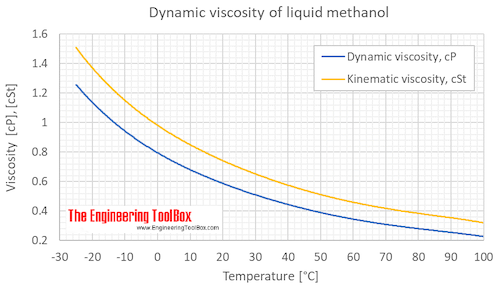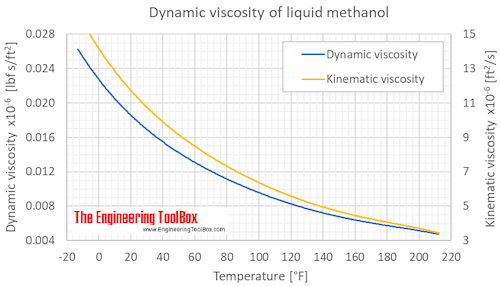Engineering ToolBox - Resources, Tools and Basic Information for Engineering and Design of Technical Applications!

# Methanol - Dynamic and Kinematic Viscosity vs. Temperature and Pressure

## Online calculator, figures and tables showing dynamic and kinematic viscosity of liquid methanol,CH3OH, at varying temperature - Imperial and SI Units.

The viscosity of a fluid is a measure of its resistance to gradual deformation by shear stress or tensile stress.

For further definitions, go to Absolute (dynamic) and kinematic viscosityAbsolute or dynamic viscosity is used to calculate Reynold's Number to determine if a fluid flow is laminar, transient or turbulent.

Tabulated values of methanol viscosity and viscosity units conversion are given below the figures.

### Online Methanol Viscosity Calculator

The calculator below can be used to estimate dynamic or kinematic viscosity of liquid methanol at given temperatures. The atmospheric boiling point of methanol is 64.15 °C (147.47 °F), and methanol must be pressurized to be present as liquid above that temperature.
The output dynamic viscosity is given as Pa*s, N*s/m2, cP, mPa*s, lbf*s/ft2 and lbm/(ft*h),
while the kinematic viscosity is given as cSt, m2/s, and ft2/s

Temperature

Choose the actual unit of temperature:

°C  °F  °R

See also other properties of Methanol at varying temperature and pressure:  Density and specific weight and Specific Heat (Heat Capacity), and Thermophysical properties at standard conditions,
as well as dynamic and kinematic viscosity of propane and water.

Dynamic and kinematic viscosity of liquid methanol at varying temperature:The atmospheric boiling point of methanol is 64.15 °C (147.47 °F), and methanol must therefore be pressurized to be present as liquid above that temperature.

Dynamic (absolute) and kinematic viscosity of liquid methanol at given temperatures and pressures, SI and Imperial units:

For full table with Dynamic and Kinematic Viscosity - rotate the screen!

 State Temperature Dynamic (Absolute) Viscosity Kinematic viscosity [K] [°C] [°F] [μPa s] [cP], [mPa s] [x10-6 lbf s/ft2 ] [x10-6 lbm s/ft s] [lbm/ft h] [cSt], [x10-6 m2/s] [x10-6ft2/s] Liquid 248.2 -25 -13 1258 1.258 0.02627 0.8453 0.003043 1.508 16.23 273.2 0.0 32 795.0 0.7950 0.01660 0.5342 0.001923 0.9815 10.56 293.2 20 68 594.0 0.5940 0.01241 0.3991 0.001437 0.7500 8.073 298.2 25 77 543.5 0.5435 0.01135 0.3652 0.001315 0.6906 7.434 303.2 30 86 507.0 0.5070 0.01059 0.3407 0.001226 0.6488 6.983 323.2 50 122 392.0 0.3920 0.00819 0.2634 0.000948 0.5142 5.535 348.2 75* 167* 294.0 0.2940 0.00614 0.1976 0.000711 0.4000 4.306 373.2 100* 212* 227.0 0.2270 0.00474 0.1525 0.000549 0.3206 3.451

* The atmospheric boiling point of methanol is 64.15 °C (147.47 °F), and methanol must therefore be pressurized to be present as liquid above that temperature.

Unit conversion:

Viscosity Dynamic, Absolute
centipoise [cP], gram/(centimeter second) [g/(cm s)] = poise [P],  kilogram/meter second [kg/m s]=newton second/square meter [N s/m2]= pascal second [Pa s], pound/(foot hour) [lb/(ft h)], pound/(foot second) [lb/(ft s)], reyn [reyn]

• 1 cP = 0.001 Pa s = 0.01 P = = 0.01 g/(cm sec) = 6.72197x10-4 lb/(ft s) = 2.4191 lb/(ft h)
• 1 lb/(ft h) = 0.00027778 lb/(ft s) = 0.00041338 Pa s = 0.0041338 P = 0.41338 cP
• 1 lb/(ft s) = 3600 lb/(ft h) = 1.48816 Pa s = 14.8816 P = 1488.16 cP
• 1 kg/(m s ) = 1 (N s)/m2 = 1 Pa s = 10 P = 1000 cP = 0.672197 lb/(ft s) = 2419.09 lb/(ft h)
• 1 (N s)/m2 = 1 kg/(m s ) = 1 Pa s = 10 P = 1000 cP = 0.672197 lb/(ft s) = 2419.09 lb/(ft h)
• 1 P = 1 g/(cm s) = 0.1 Pa s = 100 cP = 0.067197 lb/(ft s) = 241.909 lb/(ft h)
• 1 Pa s = 1 kg/(m s) = 1 (N s)/m2 = 10 P = 1000 cP = 0.672197 lb/(ft s) = 2419.08 lb/(ft h) =  0.00014504 reyn
• 1 reyn = 6894.76 Pa s

Absolute or Dynamic Viscosity Unit Converter

Viscosity Kinematic
centistoke [cSt] = square millimeter/second [mm2/s], square foot/hour [ft2/h], square foot/second [ft2/s], square inch/second [in2/s], square meter/hour [m2/h], square meter/second [m2/s], stoke [St] = square centimeter/second [cm2/s]

• 1 cm2/s = 1 St = 100 mm2/s = 100 cSt = 1x10-4 m2/s = 0.36 m2/h = 1.07639x10-3 ft2/s = 3.875008 ft2/h = 0.1550003 in2/s
• 1 cSt = 1 mm2/s = 0.01 St = 1x10-6 m2/s = 0.0036 m2/h = 1.07639x10-5 ft2/s = 0.03875008 ft2/h = 0.001550003 in2/s
• 1 ft2/h = 2.7778x10-4 ft2/s = 0.04 in2/s = 2.58064x10-5 m2/s = 0.09290304 m2/h = 25.8064 cS = 0.258064 St
• 1 ft2/s = 3600 ft2/h = 144 in2/s = 0.09290304 m2/s = 334.451 m2/h = 92903.04 cSt = 929.0304 St
• 1 in2/s = 0.0069444 ft2/s = 25 ft2/h = 0.00064516 m2/s = 2.322576 m2/h = 645.16 cSt = 6.4516 St
• 1 m2/h = 1/3600 m2/s = 2.7778x10-4 m2/s = 2.7778 cm2/s = 277.78 mm2/s = 277.78 cSt = 2.7778 St = 0.00298998 ft2/s = 10.7639 ft2/h = 0.430556 in2/s
• 1 m2/s = 3600 m2/h = 1x104 cm2/s = 1x104 St = 1x106 mm2/s = 1x106 cSt = 10.7639 ft2/s = 38750.08 ft2/h = 1550003 in2/s
• 1 mm2/s = 1 cSt = 1x10-6 m2/s = 0.0036 m2/h = 0.01 cm2/s = 0.01 St = 1.07639x10-5 ft2/s = 0.03875008 ft2/h = 0.001550003 in2/s
• 1 St = 1 cm2/s = 100 cSt = 100 mm2/s = 1x10-4 m2/s = 0.36 m2/h = 1.076x10-3 ft2/s = 3.875008 ft2/h = 0.1550003 in2/s

## Related Topics

• ### Fluid Mechanics

The study of fluids - liquids and gases. Involving velocity, pressure, density and temperature as functions of space and time.
• ### Material Properties

Material properties of gases, fluids and solids - densities, specific heats, viscosities and more.
• ### Thermodynamics

Work, heat and energy systems.
• ### Viscosities

Viscosities of products and chemical species at varying conditions.

## Related Documents

• ### Absolute or Dynamic Viscosity Online Converter

Convert between dynamic or absolute viscosity units - Poiseuille, Poise, centPoise and more.
• ### Alcohols and Carboxylic Acids - Physical Data

Molweight, melting and boiling point, density, pKa-values, as well as number of carbon and hydrogen atoms in molecules are given for 150 different alcohols and acids.
• ### Ammonia - Dynamic and Kinematic Viscosity vs. Temperature and Pressure

Online calculator, figures and tables showing dynamic (absolute) and kinematic viscosity of gasous and liquid ammonia at temperatures ranging from -73 to 425°C (-100 to 800°F) at pressure ranging from 1 to 1000 bara (14.5 - 14500 psia) - SI and Imperial Units.
• ### Benzene - Dynamic and Kinematic Viscosity vs. Temperature and Pressure

Online calculator, figures and table showing dynamic and kinematic viscosity of benzene, C6H6, at varying temperature and pressure - Imperial and SI Units.
• ### Butane - Dynamic and Kinematic Viscosity vs. Temperature and Pressure

Online calculators, figures and tables with dynamic and kinematic viscosity of liquid and gaseous butane, C4H10, at varying temperarure and pressure, SI and Imperial units.
• ### Carbon Dioxide - Dynamic and Kinematic Viscosity vs. Temperature and Pressure

Online calculator, figures and table showing dynamic and kinematic viscosity of carbon dioxide, CO2, at varying temperature and pressure - Imperial and SI Units.
• ### Ethane - Dynamic and Kinematic Viscosity

Online calculator, figures and tables showing dynamic and kinematic viscosity of ethane, C2H6, at varying temperature and pressure - Imperial and SI Units
• ### Ethanol - Dynamic and Kinematic Viscosity vs. Temperature and Pressure

Online calculator, figures and tables showing dynamic and kinematic viscosity of ethanol, C2H5OH, at varying temperature and pressure - Imperial and SI Units.
• ### Ethylene - Dynamic and Kinematic Viscosity vs. Temperature and Pressure

Online calculator, figures and tables showing dynamic and kinematic viscosity of ethylene, C2H4, also called ethene or acetene, at varying temperature and pressure - Imperial and SI Units.
• ### Freeze Protection of Water based Heat Transfer Fluids

Comparing antifreezes used in water based heat transfer fluids or brines.
• ### Hydrocarbons - Autoignition Temperatures and Flash Points

Autoignition temperatures and flash points (°C and °F) of different types of hydrocarbons with varying carbon numbers up to C12.
• ### Hydrocarbons, Alcohols and Acids - Boiling points

Boiling temperatures (°C and °F) with varying carbon numbers up to C33.
• ### Hydrocarbons, Linear Alcohols and Acids - Densities

Density of hydrocarbons like alcohols and acids as function of carbon number at 20°C / 68°.
• ### Kinematic Viscosity - Online Converter

Convert between kinematic viscosity units like centistokes, poise, lentor and more.
• ### Liquids - Latent Heat of Evaporation

Latent heat of vaporization for fluids like alcohol, ether, nitrogen, water and more.
• ### Methane - Dynamic and Kinematic Viscosity vs. Temperature and Pressure

Online calculator, figures and tables showing dynamic and kinematic viscosity of methane, CH4, at varying temperature and pressure - Imperial and SI Units.
• ### Methanol - Density and Specific Weight vs. Temperature and Pressure

Online calculator, figures and tables showing density and specific weight of methanol,CH3OH, at varying temperature and pressure - Imperial and SI Units.
• ### Methanol - Thermophysical Properties

Chemical, physical and thermal properties of methanol, CH3OH (also called carbinol, wood alcohol, hydroxy methyl and methyl alcohol). Phase diagram included.
• ### Methanol - Thermophysical Properties vs. Temperature

Thermophysical properties of methanol.
• ### Methanol Freeze Protected Heat Transfer Liquids

Freezing and flash points for methanol or methyl based heat-transfer fluids or brines.
• ### Nitrogen - Dynamic and Kinematic Viscosity vs. Temperature and Pressure

Online calculator, figures and tables showing dynamic and kinematic viscosity of nitrogen, N2, at varying temperature and pressure - Imperial and SI Units.
• ### Oxygen - Dynamic and Kinematic Viscosity vs. Temperature and Pressure

Online calculator, figures and tables showing dynamic and kinematic viscosity of oxygen, O2, at varying temperature and pressure - Imperial and SI Units.
• ### PE Polyethylene - Chemical Resistance

Chemical resistance of Polyethylene - PEH - to common products.
• ### Phenols, alcohols and carboxylic acids - pKa values

For oxygen containing organic compounds this is given: pKa (the negative logarithm of the acid dissociation constant), molecular structures, molar weights, density and melting and boiling points.
• ### Propane - Dynamic and Kinematic Viscosity vs. Temperature and Pressure

Online calculators, figures and tables showing dynamic and kinematic viscosity of liquid and gaseous propane at varying temperarure and pressure, SI and Imperial units.
• ### Reynolds Number

Introduction and definition of the dimensionless Reynolds Number - online calculators.
• ### Viscosity - Absolute (Dynamic) vs. Kinematic

Vicosity is a fluid's resistance to flow and can be valued as dynamic (absolute) or kinematic.
• ### Water - Dynamic (Absolute) and Kinematic Viscosity vs. Temperature and Pressure

Free online calculator - figures and tables with viscosity of water at temperatures ranging 0 to 360°C (32 to 675°F) - Imperial and SI Units.

## Engineering ToolBox - SketchUp Extension - Online 3D modeling!

Add standard and customized parametric components - like flange beams, lumbers, piping, stairs and more - to your Sketchup model with the Engineering ToolBox - SketchUp Extension - enabled for use with older versions of the amazing SketchUp Make and the newer "up to date" SketchUp Pro . Add the Engineering ToolBox extension to your SketchUp Make/Pro from the Extension Warehouse !

We don't collect information from our users. More about

## Citation

• The Engineering ToolBox (2018). Methanol - Dynamic and Kinematic Viscosity vs. Temperature and Pressure. [online] Available at: https://www.engineeringtoolbox.com/methanol-dynamic-kinematic-viscosity-temperature-pressure-d_2093.html [Accessed Day Month Year].

Modify the access date according your visit.

9.19.12